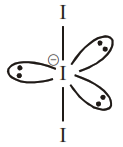# The correct shape and I-I-I bond angles respectively`
Question:

The correct shape and I-I-I bond angles respectively in $\mathrm{I}_{3}^{-}$ion are :-

1. Distorted trigonal planar; $135^{\circ}$ and $90^{\circ}$

2. T-shaped; $180^{\circ}$ and $90^{\circ}$

3. Trigonal planar; $120^{\circ}$

4. Linear; $180^{\circ}$

Correct Option: 4,

Solution:Shape : Linear, I-I-I Bond angle $\Rightarrow 180$